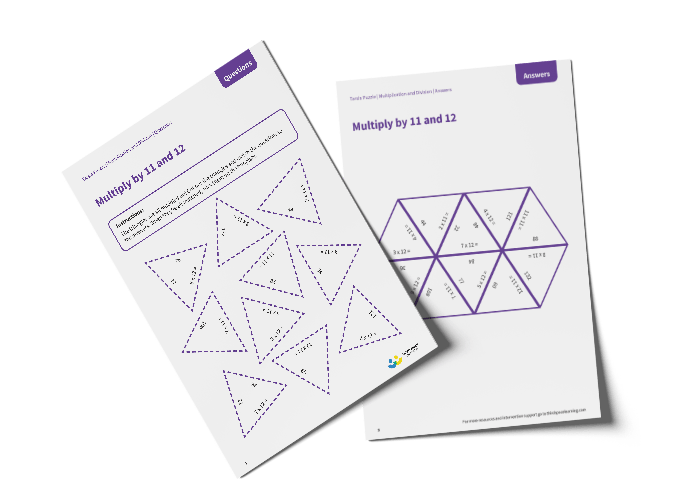# Tarsia Puzzle Multiply by 11 and 12 (Year 4)

The triangles in the tarsia puzzle have all been mixed up. In this multiplying by 11 and 12 tarsia puzzle, pupils will need to match the multiplication question to the correct answer to create one large hexagon.

This Year 4 multiply by 11 and 12 tarsia puzzle worksheet contains:

• One question sheet
• One template sheet

Year 4

Multiplication